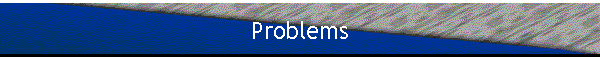Problem:

Find the condition that must be satisfied by the spherically symmetric square well potential
V(r) = -|V0|   for r < a,  V(r) = 0   for r > a,
if it is just barely deep enough to contain one bound state.

Solution:

• Concepts:
Three-dimensional square potentials
• Reasoning:
We have to investigate the properties of the ground state in a three dimensional square potential.
• Details of the calculation:
If the potential only supports one bound state, this state must be an l = 0 state.  The radial Schroedinger equation then isLet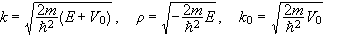Rename a = r0.
Then from a previous problem we have |sinkr0| = k/k0, in regions where cotkr0  < 0.
If we plot f(k) = |sinkr0| and g(k) = k/k0 we find solutions at the intersections of the two curves in regions where cotkr0 < 0 , i.e.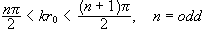To only support one bound state we need π/2 < k0r0 < 3π/2,
With E near zero we have k ~ k0 and  π/2 ~(2μV02)1/2r0.
The smallest possible value for V0 is V0 = π2ħ2/(8μr02).

Problem:

A particle of mass m is bound in a 2-dimensional isotropic oscillator potential with a spring constant k.  Use the fact that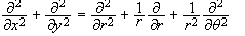,

and write the Schroedinger equation for this system in both Cartesian and polar coordinates.  Separate the equation in polar coordinates and solve the resulting equation in θ.  Write the resulting radial equation utilizing the θ solution, but do not solve it.  Demonstrate the connection between the θ solution and its classical analog.

the θ solution and its classical analog.

Solution:

• Concepts:
The Schroedinger equation in polar coordinates, separation of variables
• Reasoning:
We are asked to write the Schroedinger equation for the system in polar coordinates and separate variables.
• Details of the calculation:
The Schroedinger equation in Cartesian coordinates is.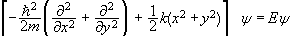.
The Schroedinger equation in polar coordinates is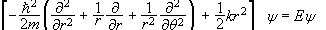.
Let ψ = f(r)χ(θ).  Then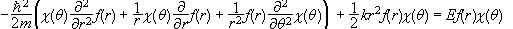.

Multiply by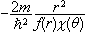.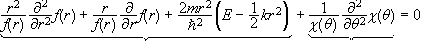.

independent of θ                independent of r
The first term is a function of r only and the second term is a function of θ only.  Both terms must be equal to a constant and the sum of these constants must be zero.since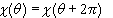.

The θ solutions are eigenfunctions of Lz with eigenvalues nħ.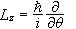commutes with H, Lz is a constant of motion, quantum mechanically as well as classically.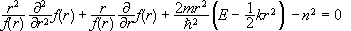.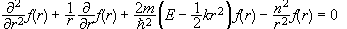.

Problem:

A particle of charge -e and mass m is under the influence of two stationary heavy nuclei, each with charge Ze positioned at  z = ±a.  We shall further assume that the particle is spinless and non relativistic.
(a)  What is the Hamiltonian and the Schroedinger equation of the system.
(b)  Define the angular momentum operator along the z - direction, Lz, and show that its eigenvalues are good quantum numbers for all of the non-degenerate energy eigenstates.  What are the possible eigenvalues of Lz?
(c)  Define the parity operator P and show that parity is a good quantum number for all the non-degenerate energy eigenstates.  What are the possible eigenvalues of P?
(d)  Define the total angular momentum operator L and show that the eigenvalues of L2 are not good quantum numbers for the energy eigenstates.

Solution:

• Concepts:
The Schroedinger equation in three dimensions, the orbital angular momentum operator, the parity operator
• Reasoning:
We are asked to write down the Schroedinger equation for the system and to define the orbital angular momentum operator and the parity operator.  We check if the eigenvalues of an operator are good quantum numbers by checking if the operator commutes with H.
• Details of the calculation:
(a)  The Hamiltonian of the electron is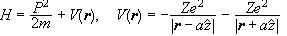.
The Schroedinger equation is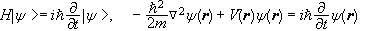.
In spherical coordinates we have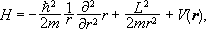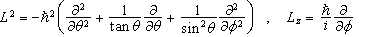,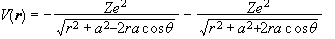.
(b)  We know [ L2,Lz] = 0, therefore [ H,Lz] = 0, since the L2 term is the only one with a φ dependence.  If two operators commute, then a common eigenbasis can be found.  An eigenstate with a non-degenerate eigenvalue of H is also an eigenstate of Lz.  The possible eigenvalues of Lz are nħ where n is an integer.
(c)  We define the parity operator through its action on any ψ(r).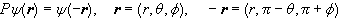.
[P,L2] = 0, since changing θ to π - θ and φ to π + φ does not change L2.
[P,V(r)] = 0.  since changing  θ to π - θ does not change V(r).
Therefore [P,H] = 0.  As above, an eigenstate with a non-degenerate eigenvalue of H is also an eigenstate of P.  The eigenvalues of P are ±1.

(d)  In spherical coordinates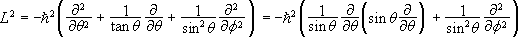.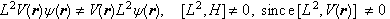.
No common eigenbasis of L2 and H exists.

Problem:

Two particles of mass m1 and m2 are separated by a fixed distance r.  Their center of mass is fixed at the origin of the coordinate system and they are free to rotate about their center of mass.  (The system is a "rigid rotator".)
(a)  Write down the Hamiltonian of the system.
(b)  Find the eigenvalues and eigenfunctions of this Hamiltonian.  What is the separation between adjacent levels?  What is the degeneracy of the eigenvalues?

Solution:

• Concepts:
The time-independent Schroedinger equation in three dimensions, the eigenfunctions of the orbital angular momentum operator.
• Reasoning:
We can write the Hamiltonian for the motion about the center of mass as that of a fictitious particle of reduced mass m moving in a central potential.  We solve the Schroedinger equation in spherical coordinates.
• Details of the calculation:
(a)  The Hamiltonian of the rotator is L2/(2I) where I is its moment of inertia,
I = m1r12 + m2r22 = μr2,  μ = m1m2/(m1 + m2).
(b) The eigenfunctions of H are the eigenfunctions of L2.
These are the spherical harmonics, Ylm(θ,φ) = <r/r|l,m>.
The eigenvalues are El = l(l + 1)ħ2/(2I).
It is customary to set  B = ħ/(4πI), and write El = Bhl( l+ 1).
The separation between adjacent levels is  El - El-1 = Bh[l(l + 1) - (l - 1)l] = 2Bhl.
The separation increases linear with l.  Each energy eigenvalue is 2l + 1 fold degenerate.